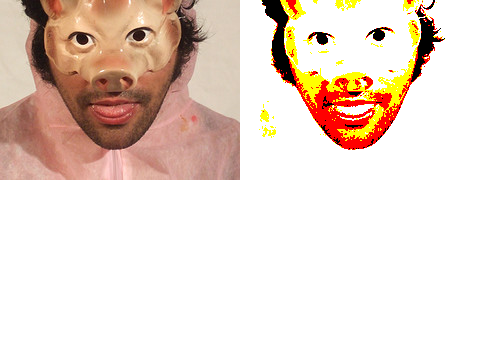# OPENCV

Name threshold()
Examples```import hypermedia.video.*;

OpenCV opencv;
size( 480, 360 );

opencv = new OpenCV( this );
image( opencv.image(), 0, 0 );                   // show original
opencv.threshold(80);                            // filtering out pixels > 80 to 255
image( opencv.image(), 240, 0 );                 // show the result

```
Description Apply fixed-level threshold to the current image.

This method applies fixed-level thresholding to single-channel array. It is typically used to get bi-level (binary) image out of grayscale image or for removing a noise (filtering out pixels with too small or too large values).

About types of thresholding

• THRESH_BINARY : dst(x,y) = src(x,y) > value ? max : 0
• THRESH_BINARY_INV : dst(x,y) = src(x,y) > value ? 0 : max
• THRESH_TRUNC : dst(x,y) = src(x,y) > value ? value : src(x,y)
• THRESH_TOZERO : dst(x,y) = src(x,y) > value ? src(x,y) : 0
• THRESH_TOZERO_INV : dst(x,y) = src(x,y) > value ? 0 : src(x,y)
• THRESH_OTSU : Use Otsu algorithm to choose the optimal threshold value, combine the flag with one of the above THRESH_* values
Syntax `threshold(value, max, type);threshold(value);`
Parameters
 value float : threshold value max float : the maximum value to use with THRESH_BINARY and THRESH_BINARY_INV thresholding types. type int : Types of thresholding
Return float
Usage Application